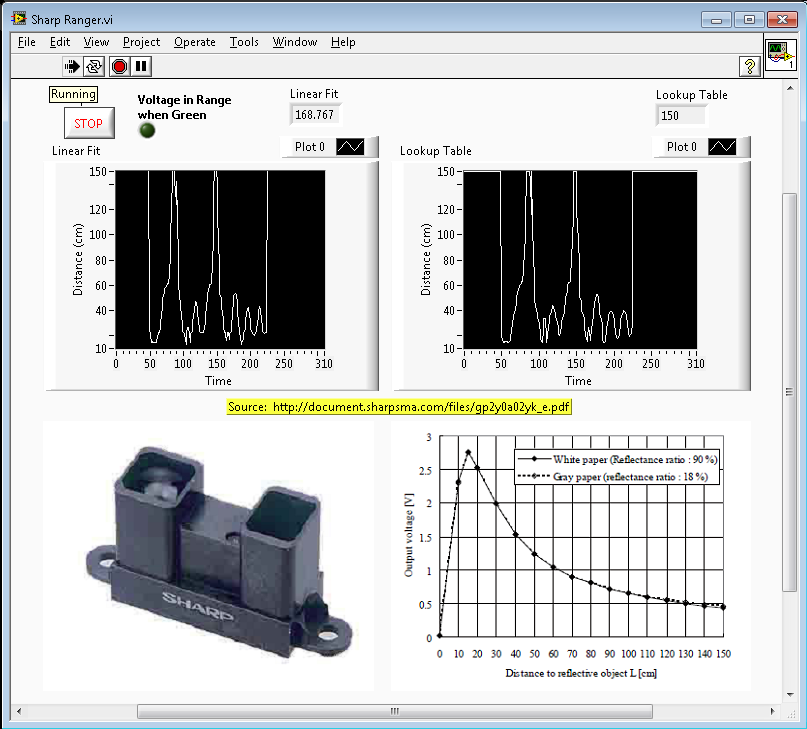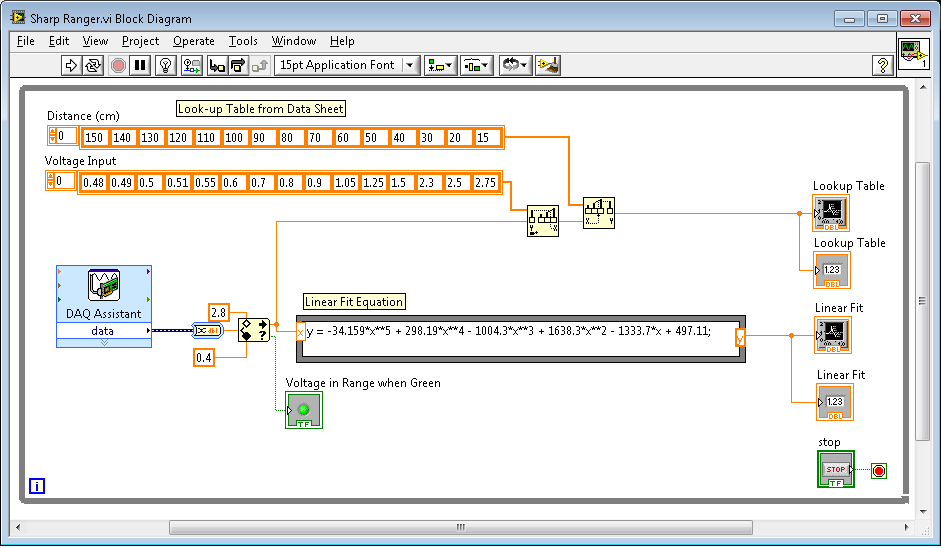# myDAQ Student Projects

cancel
Showing results for
Did you mean:

## Sharp IR Ranging Sensor on NI myDAQ

The Sharp IR Ranger (GP2Y0A02YK) uses infrared reflection to detect distances from about 15cm to 150cm.  The DAQ Assistant is used in the attached VI to acquire voltage values from the range finder.  Then two methods (a lookup table and 5th order linear fit equation) are used to estimate the actual distance of the object from the sensor.

Sensor:

Wiring Instructions:

The sensor uses 3 wires: red (+5), black (Ground), and yellow (Input signal).  The red wire should be wired to the +5 input on myDAQ, the black wire to ground, and the yellow wire to AI1+.  The final connection is a short wire to jumper from ground to AI1-.

The LabVIEW VI

The attached VI (Sharp Ranger.vi) front panel contains images from the data sheet as well as two strip charts.  Each chart shows the detected distnace.As seen from the bock diagram, the DAQ assistant is used to acquire analog voltages from AI1, limited to the voltage ranges using a thresholding function, and run through both a linear fit and lookup table operations to determine the measured distance.Calculating the Range:

Calculating the range is kind of tricky, because the voltage does not linearly correspond to distance.  The lookup table is roughly given from the data sheet.  Using a I plotted those values and calculated a linear fit equation.  From there I used a Formula Node to caculate the corresponding distance.  (keep in mind that the linear fit would produce very odd values at both the high and low extremes if I had not added the thresholding VI to the input signal prior to the calculation.